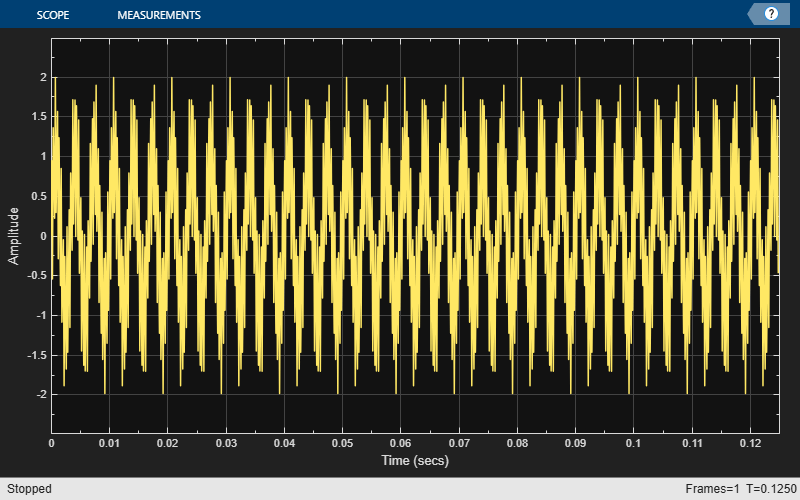# hide

Hide scope window

## Syntax

``hide(scope)``

## Description

````hide(scope)` hides the scope window.```

## Examples

collapse all

Create a time-domain sinusoidal signal. Display the signal by calling the time scope object.

Create a sinusoidal signal with two tones, one at 0.3 kHz and the other at 3 kHz.

```t = (0:1000)'/8e3; xin = sin(2*pi*0.3e3*t)+sin(2*pi*3e3*t);```

Create a `timescope` object and view the sinusoidal signal by calling the time scope object `scope`.

```scope = timescope(SampleRate=8e3,... TimeSpanSource="property",... TimeSpan=0.1); scope(xin)```Run `release` to allow changes to property values and input characteristics. The scope automatically scales the axes.

`release(scope);`Hide the scope window.

```if(isVisible(scope)) hide(scope) end```

Show the scope window.

```if(~isVisible(scope)) show(scope) end```## Input Arguments

collapse all

Scope object whose window you want to hide, specified as a `timescope` object.

Example: ```myScope = timescope; hide(myScope)```

## Version History

Introduced in R2020a